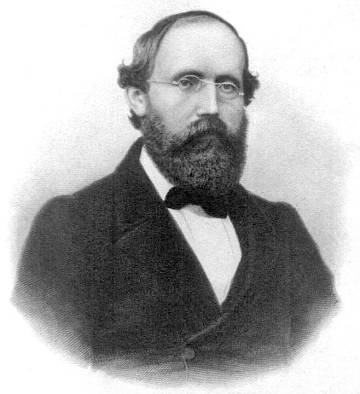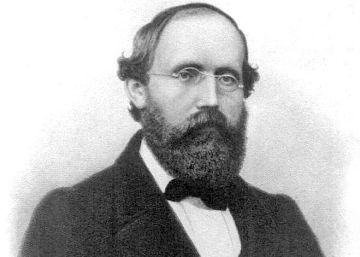Fri. Sep 20th, 2019

# A mathematician linked to an enigmatic hypothesis | ScienceAnyone with a minimum interest in mathematics will have heard of the Riemann hypothesis, one of the biggest unsolved problems of discipline. However, how many know the mathematician behind this statement? With the bibliometric indexes that are used so much today to evaluate scientists, it would undoubtedly criticize Bernhard Riemann (1826-1866) its scarce production (even considering that he died young) but his enormous impact would be praised. There are the Riemann metrics, the Riemann tensor, the Riemann application theorem and many other concepts that honor his name.

Among his works, perhaps he who has given more than talk is a very brief memory published in 1859, which includes his famous hypothesis. There you enter a function, called ζ, defined on complex numbers. The surprising thing is that this function contains information about the distribution of prime numbers. Riemann achieves with analytical techniques (derived and integral) to express the quantity of prime numbers smaller than an arbitrary value, in terms of the values ​​in which ζ is annulled (that is, where 0 is worth).

It is known that ζ is canceled in the negative pairs (-2, -4, -6, ...), which turn out to contribute little to the account of the cousins, however, the rest of the values ​​in which ζ is canceled called non-trivial zeros, are much more mysterious and give rise to oscillatory terms, waves. In this way the cousin count is written as a superposition of waves associated with non-trivial zeros. It turns out that the amplitude of these waves is linked to the distance to a certain straight line. If all non-trivial zeros were in that line, very precise estimates would be obtained about the distribution of the cousins. But is it true that this is the provision?

Riemann's hypothesis assures that yes: non-trivial zeros are in Indian rank, but the spacing is variable, it happens as in the tail of a great event: the gap between two consecutive people varies depending on whether they are friends or not but always, as the line advances towards its destination the spaces are reduced. In the same way, when the non-trivial zeros of the function are traversed ζ they become more crowded, although the precise spacing, the friendship between zeros, seems to be regulated by the laws of chaos in a way that has interested theoretical physicists and of course mathematicians.

In case it was not rare enough that the zeros of the function introduced by Riemann are aligned for no apparent reason, the phenomenon has an incredible ubiquity. Thus there is a plethora of functions, generically called L functions, which appear in very different subjects (for example in the acclaimed proof of Fermat's last theorem) and which also seem to satisfy Riemann's hypothesis.

All passionate about numbers hope to see in a future birthday of Riemann the great gift that would be the test of his hypothesis. If the step to posterity were not enough incentive, the foundation Clay Mathematics Institute offers a million dollars for it

Atle Selberg, one of the great specialists in the ζ function of the twentieth century, made a risky guess about it. In vague terms (the person interested in something more precise can look for information on the "Selberg class"), he surmised that the Riemann hypothesis is fulfilled for any function L as long as it is defined for complex numbers, it has coefficients that grow little bit, satisfy some symmetry and be related to factoring.

Incidentally, Selberg owes one of the greatest advances related to the Riemann hypothesis, showed that a part of the zeros are in the expected line. Currently it's known that more than 40% of them are. Other Outcome much more simple and classic, but also notable, is that even if Riemann's hypothesis were false, the vast majority of non-trivial zeros should be concentrated very close to the line.

Riemann's hypothesis admits innumerable consequences and equivalences, some of a very elementary nature, for example, related to divisor sums (robin's theorem), to irreducible fractions (Franel's theorem) or calculation of determinants (redheffer matrix).

All passionate about numbers hope to see in a future birthday of Riemann (September 17) the great gift that would be the test of his hypothesis. If the step to posterity were not enough incentive, the foundation Clay Mathematics Institute offers a million dollars for it.

Fernando Chamizo He is a professor at the Autonomous University of Madrid and a member of ICMAT.

Agate Timón García-Longoria He is responsible for Communication and Disclosure of ICMAT.

Coffee and theorems It is a section dedicated to mathematics and the environment in which they are created, coordinated by the Institute of Mathematical Sciences (ICMAT), in which researchers and members of the center describe the latest advances in this discipline, share meeting points between mathematics and other social and cultural expressions and remember those who marked their development and knew how to transform coffee into theorems. The name evokes the definition of the Hungarian mathematician Alfred Rényi: "A mathematician is a machine that transforms coffee into theorems."

Editing and coordination: Agate Timon (ICMAT).

You can follow Matter in Facebook, Twitter, Instagram or subscribe here to our newsletter

. (tagsToTranslate) mathematician (t) link (t) enigmatic (t) enigmatic (t) hypothesis (t) German (t) bernhard riemann (t) publish (t) 1859 (t) brief (t) memory (t) famous (t) famous (t) bear (t) name (t) consider (t) large (t) mystery (t) math